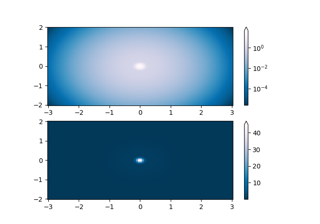# matplotlib.colors.TwoSlopeNorm¶

class matplotlib.colors.TwoSlopeNorm(vcenter, vmin=None, vmax=None)[source]

Normalize data with a set center.

Useful when mapping data with an unequal rates of change around a conceptual center, e.g., data that range from -2 to 4, with 0 as the midpoint.

Parameters: vcenterfloatThe data value that defines 0.5 in the normalization. vminfloat, optionalThe data value that defines 0.0 in the normalization. Defaults to the min value of the dataset. vmaxfloat, optionalThe data value that defines 1.0 in the normalization. Defaults to the the max value of the dataset.

Examples

This maps data value -4000 to 0., 0 to 0.5, and +10000 to 1.0; data between is linearly interpolated:

>>> import matplotlib.colors as mcolors
>>> offset = mcolors.TwoSlopeNorm(vmin=-4000.,
vcenter=0., vmax=10000)
>>> data = [-4000., -2000., 0., 2500., 5000., 7500., 10000.]
>>> offset(data)
array([0., 0.25, 0.5, 0.625, 0.75, 0.875, 1.0])

__call__(self, value, clip=None)[source]

Map value to the interval [0, 1]. The clip argument is unused.

__init__(self, vcenter, vmin=None, vmax=None)[source]

Normalize data with a set center.

Useful when mapping data with an unequal rates of change around a conceptual center, e.g., data that range from -2 to 4, with 0 as the midpoint.

Parameters: vcenterfloatThe data value that defines 0.5 in the normalization. vminfloat, optionalThe data value that defines 0.0 in the normalization. Defaults to the min value of the dataset. vmaxfloat, optionalThe data value that defines 1.0 in the normalization. Defaults to the the max value of the dataset.

Examples

This maps data value -4000 to 0., 0 to 0.5, and +10000 to 1.0; data between is linearly interpolated:

>>> import matplotlib.colors as mcolors
>>> offset = mcolors.TwoSlopeNorm(vmin=-4000.,
vcenter=0., vmax=10000)
>>> data = [-4000., -2000., 0., 2500., 5000., 7500., 10000.]
>>> offset(data)
array([0., 0.25, 0.5, 0.625, 0.75, 0.875, 1.0])

__module__ = 'matplotlib.colors'
__slotnames__ = []
autoscale_None(self, A)[source]

Get vmin and vmax, and then clip at vcenter

## Examples using matplotlib.colors.TwoSlopeNorm¶